Courses

# Differential Equations - 12

## 20 Questions MCQ Test Topic-wise Tests & Solved Examples for IIT JAM Mathematics | Differential Equations - 12

Description
This mock test of Differential Equations - 12 for Mathematics helps you for every Mathematics entrance exam. This contains 20 Multiple Choice Questions for Mathematics Differential Equations - 12 (mcq) to study with solutions a complete question bank. The solved questions answers in this Differential Equations - 12 quiz give you a good mix of easy questions and tough questions. Mathematics students definitely take this Differential Equations - 12 exercise for a better result in the exam. You can find other Differential Equations - 12 extra questions, long questions & short questions for Mathematics on EduRev as well by searching above.
QUESTION: 1

### The solution of the differential equation yy' + y2 - x = 0, where c is a constant, is

Solution: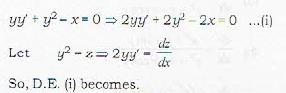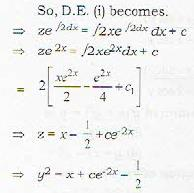QUESTION: 2

### Let k be a real constant. The solution of the differential equations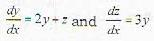satisfies the relation

Solution: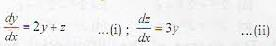Differentiating equation (i) w.r.t. we get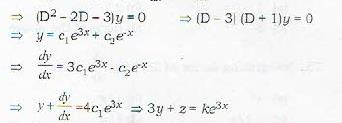QUESTION: 3

### If general solution of the differential equation ay",+ by"- cy’+ dy = 0 is linearly spanned by ex sin x and cos x, then which one of the following holds?

Solution:

Linearly independent solutions of ay" + by”+cy' + dy = 0 are ex cos x and sin x, so roots of aD3 + bD2 + cD + d = 0 will be 1 and ±f, so we have 1 +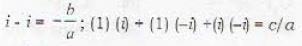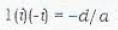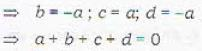QUESTION: 4

Two linearly independent solutions of the differential equation y" - 2y' + y = 0 are y1 = ex and y2 = xex. Then a particular solution of y2 - 2y' + y + ex sin x is

Solution:

Particular integral of y" - 2y' + y - ex sin x is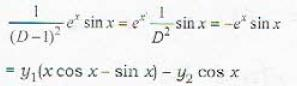QUESTION: 5

Orthogonal trajectories of the family of curves (x - 1)2 + y2 + 2ax = 0 are the solutions of the differential equation

Solution: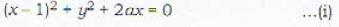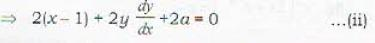By putting the value of a from equation (ii) in equation (i), we get.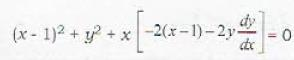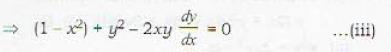It ’s orthogonal trajectory will be D.E.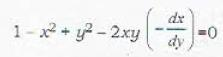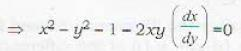QUESTION: 6

Which one of the following differential equations represents all circles with radius a?

Solution:

Equation of the circles with radius ‘a' is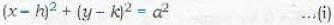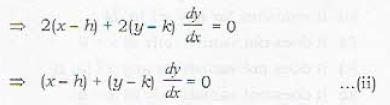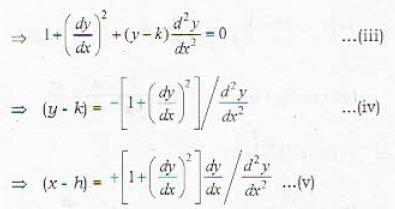Putting values of (y - k) and (x - h) from equation (iv) and equation (v) in equation (i), we get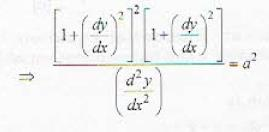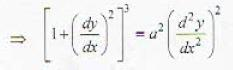QUESTION: 7

The solution of the differential equation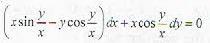with initial condition y(0) = 0 is

Solution: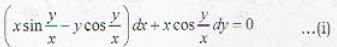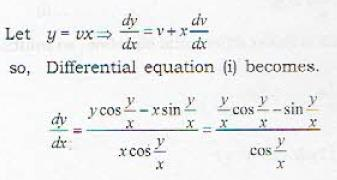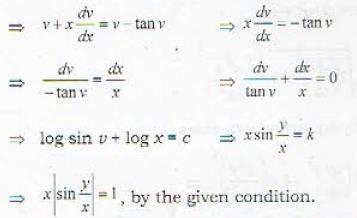QUESTION: 8

The differential equation (2a2 + by2) dx + cxydy = 0 is made exact by multiplying the integrating factor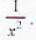Then the relation between b and c is.

Solution:

Integrating factor of (2x2 + by2) dx + cxy dy = 0 is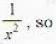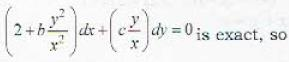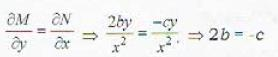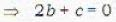QUESTION: 9

Given that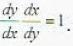Which one of the following is always true?

Solution: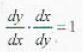....(i)
Differentiating both sides w.r.t. x, we get.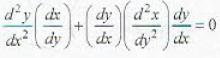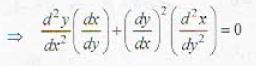QUESTION: 10

The sum of the intercepts made on the axes of co-ordinates by any tangent to the curve √x + √y = 2 is equal to

Solution: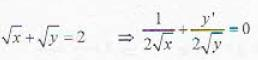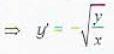Equation of tangent is (Y - y) = y'(X - x)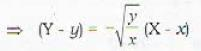Intercept on Y-axis.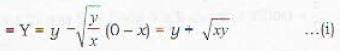Intercept on X-axis: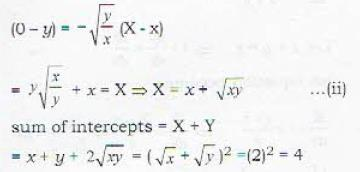QUESTION: 11

Solution of the equation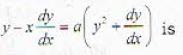Solution:

0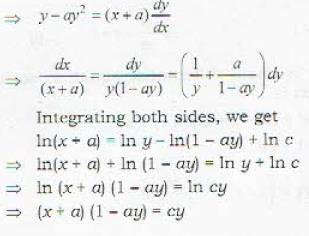QUESTION: 12

If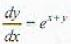and it is known that for a = 1, y = 1; if x = -1, then the value of y will be :

Solution: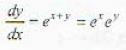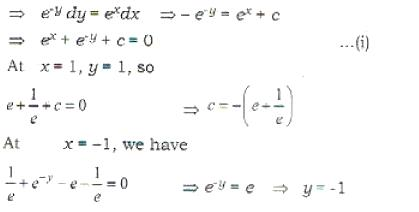QUESTION: 13

The solution of the differential equation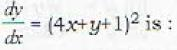Solution: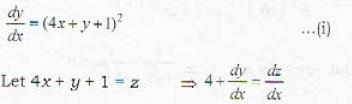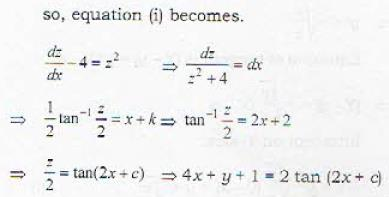QUESTION: 14

If the solution of the differential equation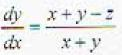is x + y -1 = Ceu then the value of u is :

Solution: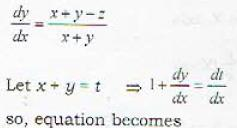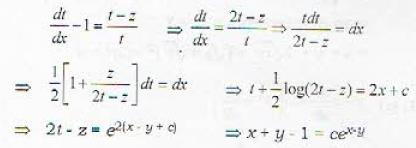QUESTION: 15

The solution of the equation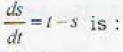Solution: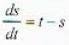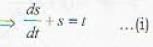which is linear differential equation so, it’s solution is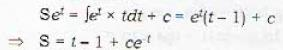QUESTION: 16

The solution of the differential equation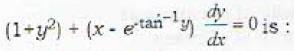Solution: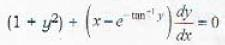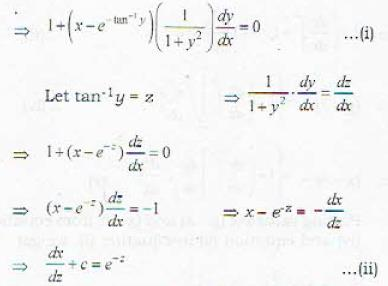which is linear differential equation in dependent variable x and independent variable z.
whose solution is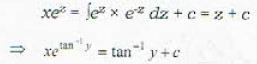QUESTION: 17

The solution of the equation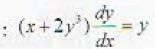Solution: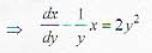.......(i)
which is linear differential equation, so solution is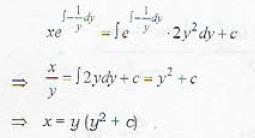QUESTION: 18

The degree and order of differential equation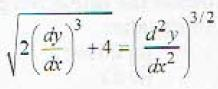are respectively

Solution: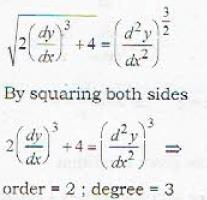QUESTION: 19

The solution of the equation (2x + y + 1)dx + (4x + 2y – 1)dy = 0 is:

Solution: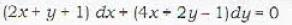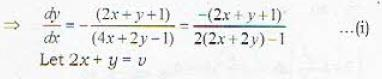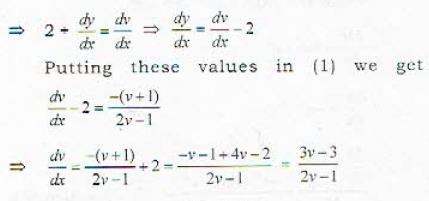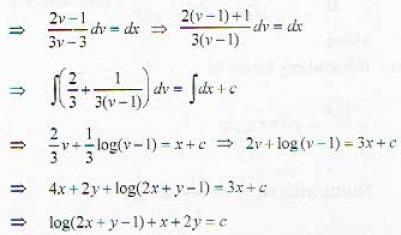QUESTION: 20

The solution of the differential equation y(x2y + ex)dx- exdy = 0 is

Solution: